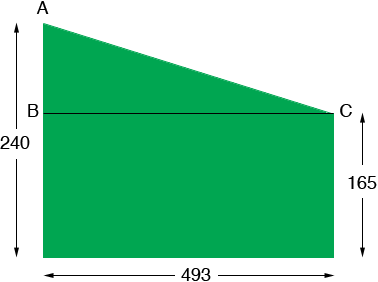SEARCH HOMEMath Central Quandaries & QueriesQuestion from Warren, a parent: The west property line is 240' long with a 90-degree angle to the south property line. The south property line is 493' long with a 90-degree angle to the east property line. The east boundary is 165' long. The length of the north property line is not known but it is a straight line from the north end of the east property line to the north end of the west property line. Could you please tell me the total square feet and how many acres? And how you calculated the answerHi Warren,

I drew a diagram of your property (not to scale) with the dimensions in feet.I drew a line CB from the north east corner to the west side so that this line is perpendicular to the west side. This partitions the property into a rectangle and a triangle. Thus the area of the property is the area of the rectangle plus the area of the triangle. The rectangle is 493 ft by 165 ft and the triangle has a base of length 493 ft and a height of 240 - 165 = 75 ft. Thus the area of your property is

area of the rectangle + area of the triangle
= length × width + 1/2 base × height
= 493 × 165 + 1/2 × 493 × 75
= 99832.5 square feet.

There are 43560 square feet in an acre so this is

99832.5/43560 = 2.3 acres.

PennyMath Central is supported by the University of Regina and The Pacific Institute for the Mathematical Sciences.